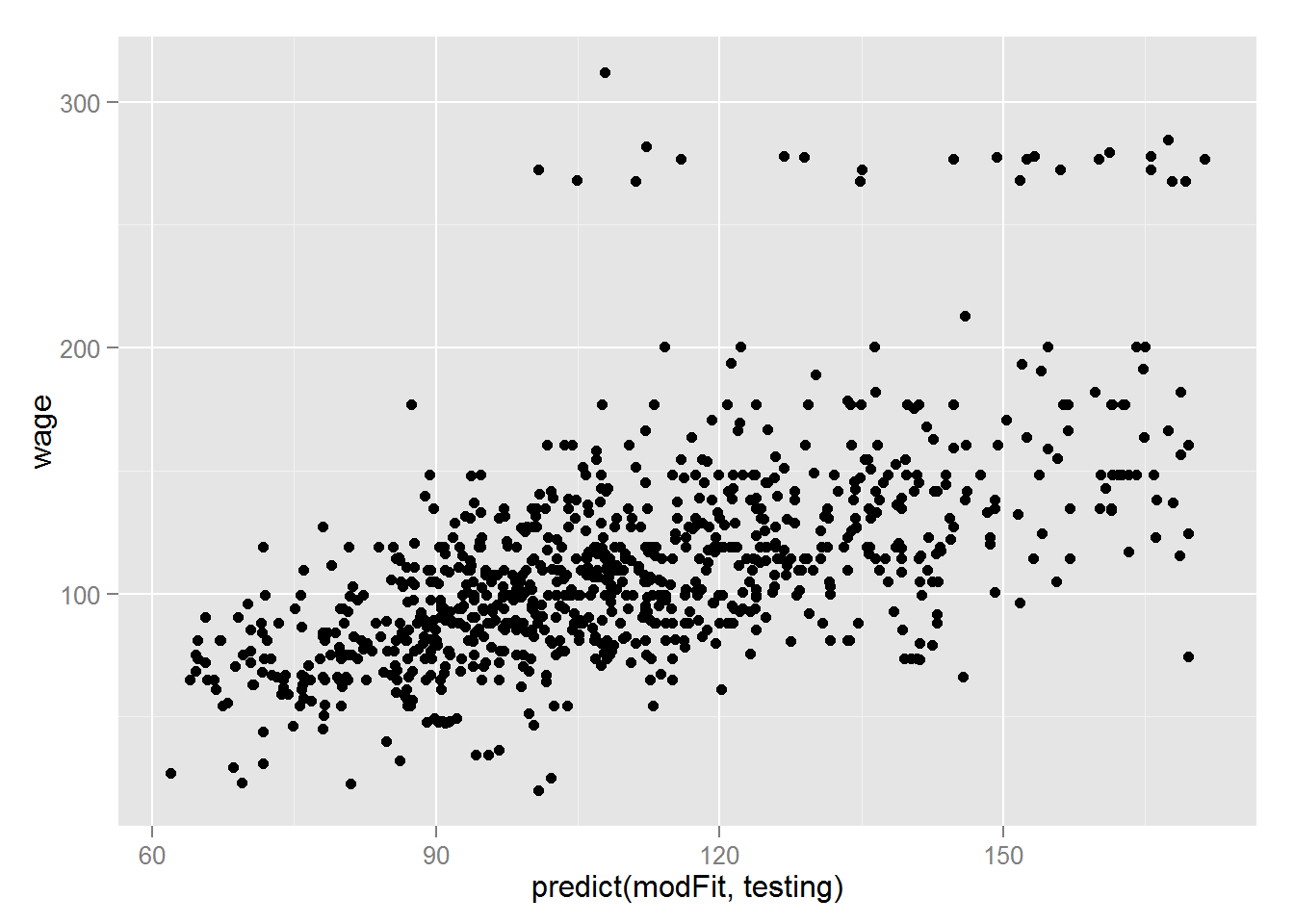Boosting is one of the bset box classifier

Basic Idea
Take lots of (poosibly) weak predictors

Weight them and add them up

Get a stronger predictor

Boosting in R
Boosting can be used with any subset of classifiers One large subclass is gradient boosting R has multiple boosting libraries. Differences include the choice of basic classification functions and combination rules. gbm - boosting with trees. mboost - model based boosting ada - statistical boosting based on additive logistic regression gamBoost for boosting generalized additive models Most of these are available in the caret package

Wage example

library(ISLR); data(Wage); library(ggplot2); library(caret);
## Loading required package: lattice
Wage <- subset(Wage,select=-c(logwage))
inTrain <- createDataPartition(y=Wage\$wage,
p=0.7, list=FALSE)
training <- Wage[inTrain,]; testing <- Wage[-inTrain,]

#Fit the model
modFit <- train(wage ~., method="gbm",data=training,verbose=FALSE)
## Loading required package: gbm
##
## Attaching package: 'survival'
##
## The following object is masked from 'package:caret':
##
##     cluster
##
## Loading required package: plyr
print(modFit)
## Stochastic Gradient Boosting
##
## 2102 samples
##   10 predictor
##
## No pre-processing
## Resampling: Bootstrapped (25 reps)
## Summary of sample sizes: 2102, 2102, 2102, 2102, 2102, 2102, ...
## Resampling results across tuning parameters:
##
##   interaction.depth  n.trees  RMSE      Rsquared   RMSE SD   Rsquared SD
##   1                   50      35.15759  0.3038776  1.530055  0.02487676
##   1                  100      34.62096  0.3137957  1.439743  0.02232634
##   1                  150      34.55009  0.3156775  1.394560  0.02166566
##   2                   50      34.60094  0.3156275  1.455682  0.02485196
##   2                  100      34.47184  0.3182034  1.374485  0.02300061
##   2                  150      34.54167  0.3162264  1.387161  0.02253147
##   3                   50      34.47797  0.3187507  1.440898  0.02512358
##   3                  100      34.59459  0.3142979  1.374172  0.02372285
##   3                  150      34.82958  0.3071383  1.363458  0.02276088
##
## Tuning parameter 'shrinkage' was held constant at a value of 0.1
##
## Tuning parameter 'n.minobsinnode' was held constant at a value of 10
## RMSE was used to select the optimal model using  the smallest value.
## The final values used for the model were n.trees = 100,
##  interaction.depth = 2, shrinkage = 0.1 and n.minobsinnode = 10.
qplot(predict(modFit,testing),wage,data=testing)#### 'MOOC > Practical Machine Learning (r programing)' 카테고리의 다른 글

 Week 04: Regularized Regression  (0) 2015.11.24 2015.11.23 2015.11.23 2015.11.23 2015.11.19 2015.11.19